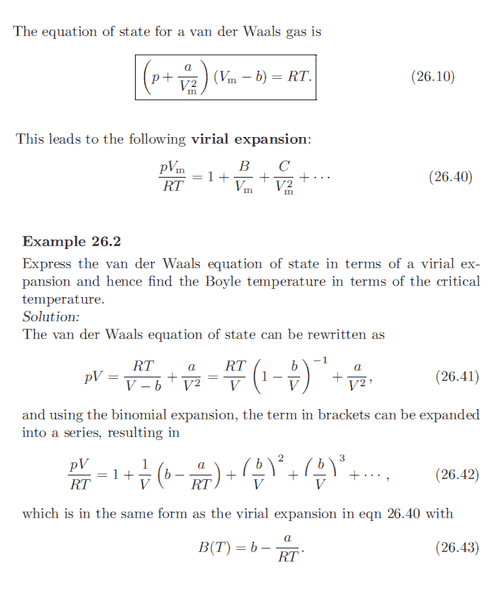# Van der Waals Equation, Virial Expansion

## Homework Statement

Taken from Concepts in thermal Physics:## The Attempt at a Solution

Shouldn't the van der waal's equation be:

$$p = \frac{RT}{V_m -b} - \frac{a}{V_m^2}$$
$$pV_m = \frac{VRT}{V_m -b} - \frac{a}{V_m}$$

## Answers and Replies

TSny
Homework Helper
Gold Member
Shouldn't the van der waal's equation be:

$$p = \frac{RT}{V_m -b} - \frac{a}{V_m^2}$$

Yes. So (26.41) has a wrong sign. But it looks like they got it right in (26.42) and (26.43).

•1 person
Chestermiller
Mentor

## Homework Statement

Taken from Concepts in thermal Physics:## The Attempt at a Solution

Shouldn't the van der waal's equation be:

$$p = \frac{RT}{V_m -b} - \frac{a}{V_m^2}$$
$$pV_m = \frac{VRT}{V_m -b} - \frac{a}{V_m}$$

You're right. It sure looks like they made a slew of algebra mistakes. The first term on the right hand side of 26.41 isn't even dimensionally correct.

Chet

•1 person
TSny
Homework Helper
Gold Member
You're right. It sure looks like they made a slew of algebra mistakes. The first term on the right hand side of 26.41 isn't even dimensionally correct.

Chet

Right. I didn't notice that they should not have had the factor of V on the left side of equation 26.41.